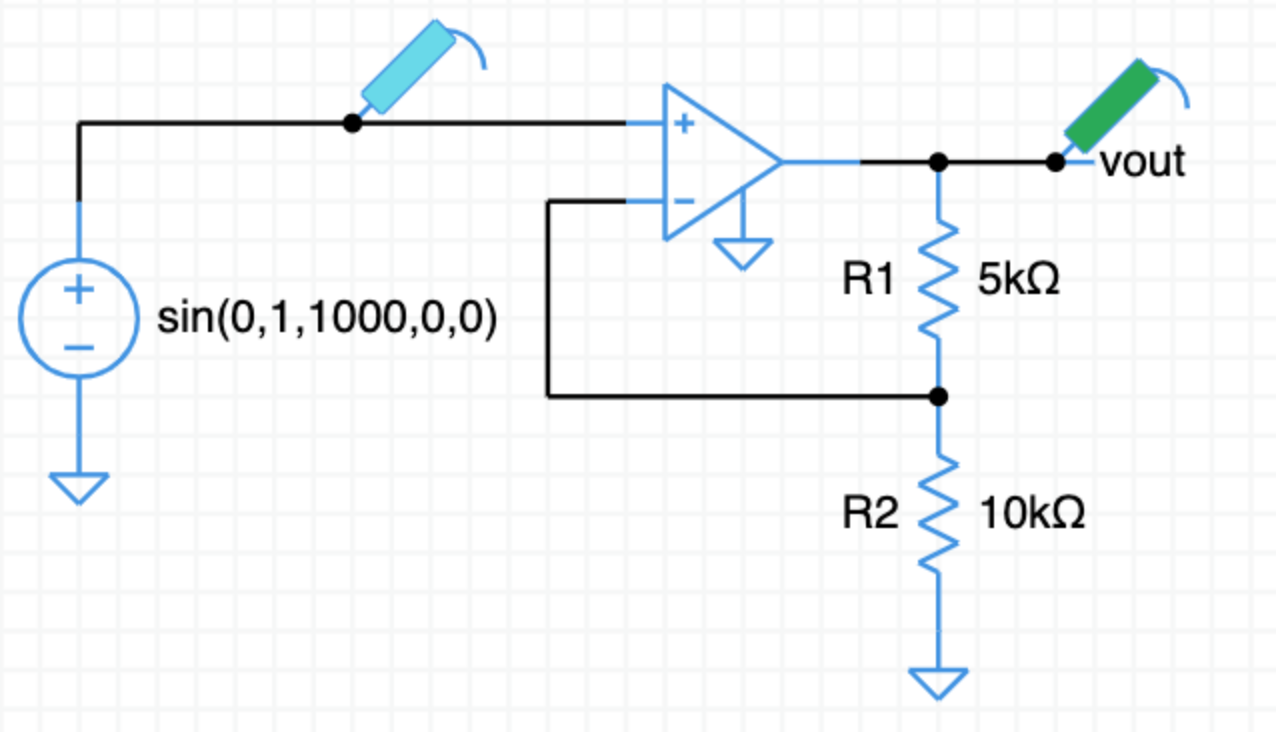What is the gain of this amplifier?

From the video, the gain expression for the non-inverting amplifier is,

$v_o = v_{in} \,\dfrac{\text R1 + \text R2}{\text R2}$

Fill in the resistor values,

$v_o = v_{in} \,\dfrac{5\,\text k + 10\,\text k}{10\,\text k}$

$v_o = v_{in} \,\dfrac{15}{10}$

$v_o = 1.5\,v_{in}$

The gain is $1.5$.

Click on TRAN to run a transient simulation.

Does the simulation match your gain calculation?

Design an amplifier with a gain of $2$.

Hint: Pick new values for either or both of $\text R1$ and $\text R2$.

Change the values of the resistors in the simulation and run TRAN again.

Verify your new amplifier has the gain you expect.

Starting from scratch with a blank schematic, design and simulate a non-inverting amplifier with a gain of $30$.

Circuit sandbox